EnchantedLearning.com is a user-supported site.
As a bonus, site members have access to a banner-ad-free version of the site, with print-friendly pages.

 +, - EnchantedLearning.comMath Dictionary x, ÷
 A B C D E F G H I J K L M N O P Q R S T U V W X Y ZA is for ...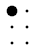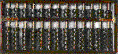abacusAn abacus is an ancient device that is used for arithmetic calculations.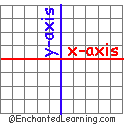abscissaThe abscissa is the x-axis of a coordinate system. The numerical value of the abscissa tells you the distance from the origin along the x-axis. The abscissa is the first number, x, in the coordinates of a point (x,y). |-3|=3absolute valueThe absolute value of a number is the distance from the origin on a number line. For example, the absolute value of 2 is 2 (written |2|=2). The absolute value of -2 is also 2 (written |-2|=2). abundant numberAn abundant number (also called a redundant number) is a positive integer for which the sum of its proper factors (divisors) is greater than the number itself. For example, 12 is an abundant number because the proper divisors of 12 are 1, 2, 3, 4, 6, which add up to 16, which is greater than 12. Compare with perfect number, deficient number.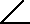acute angleAn acute angle is less than 90 degrees.acute triangleAll three interior angles of an acute triangle are acute (less than 90 degrees).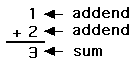addendThe addend is one of the numbers that is being added in order to find a sum. 1+1=22+3=5additionAddition is the summing of numbers to determine their total.
 0additive identityThe additive identity is the number zero because zero plus any number is equal to the original number. For example, 2+0=0+2=2. algebraAlgebra is the study of generalized arithmetic. In algebra, unknown numbers can be represented by letters in order to solve equations. For example, 4 + x = 10 is true for x=6. Algebra (originally called al-jabr, meaning "restoration") was invented in the Middle East by Abu Ja'far Muhammad ibn Musa Al-Khwarizmi (born in Baghdad about AD 825) during the Middle Ages. algorithmAn algorithm is series of steps or rules used for solving a problem. For example, the standard method for doing long division problems is an algorithm.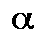alphaAlpha is the first letter in the Greek alphabet. Alpha often denotes the first term in a series or the brightest star in a constellation.angleAn angle is the amount of rotation it would take to put one intersecting line on top of another. AngstromAn Angstrom is equal to one ten billionth (1 x 10-10) of a meter. This unit of measure is named for the Swedish physicist Anders J. Ångström. angular distanceAngular distance is the measure of an arc (a segment of the circumference of a circle). Angular distance measures the proportion of a circle that the arc in question consists of. Angular distance is expressed in degrees, radians, arc minutes (one-sixtieth of a degree), or arc seconds (one-sixtieth of a minute). For example, latitude is the angular distance north or south of the equator measured in degrees, and longitude is the angular distance east or west of the prime meridian (which goes through Greenwich, England), measured in degrees. arcAn arc is a segment along the circumference of a circle, or the part of any curve between two points.
Figure Area
Square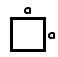a * a
Rectanglea * b
Circle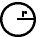πr 2

area

The area of a region is the number of square units contained within the region. For example, the area of a square with a sides of length a is A = a2. The area of a rectangle is A = length*width. The area of a parallelogram is A = base*height. The area of a triangle is (1/2)base*height. The area of a circle is A = >πr2
1+1=2

7-4=3

arithmetic

Arithmetic is the study of addition, subtraction, multiplication, and division.

arithmetic mean

The arithmetic mean of a set of numbers (also called the average) is equal to the sum of the numbers divided by the number of numbers. For example, for the data set {1, 2, 3, 6}, the mean is (1+2+3+6)/4 = 12/4 = 3.

arithmetic sequence (or arithmetic progression)

An arithmetic sequence, also called an arithmetic progression, is an ordered list of numbers where each term is obtained by adding (or subtracting) a constant amount to the previous term. For example, the arithmetic sequence
0, 2, 4, 6, 8, 10, ...
is the sequence of numbers starting with 0 where the terms increase by 2 in turn.
 (a + b) + c =a+ (b + c)(a x b) x c =a x (b x c)associative propertyThe associative property for an operation states that changing the grouping of the numbers does not change the result of the operation. For example, addition and multiplication have the associative property. Subtraction and division do not have the associative property.asymmetricalSomething that is asymmetrical is not symmetrical - it does not have symmetry. For example, the figure above is asymmetrical.asymptoteAn asymptote is a straight line that defines the limits of a curve (such as a hyperbola). In the picture above, the dashed lines are asymptotes of the hyperbola. averageAlso called the arithmetic mean, the average of of a set of numbers is the sum of the numbers divided by the number of numbers. For example, the average of 1,4,6,9 is (1+4+6+9)/4=5.
 axiomAn axiom is a statement that is assumed or accepted to be true as a starting point for proving other things.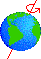axisAn axis is an imaginary straight line around which an object, like a planet, turns. For example, the Earth's axis is a line that goes through the North and South Poles. axis of symmetryAn axis of symmetry is a line about which something is symmetrical. For example, the diameter of a circle is an axis of symmetry.

 +, - EnchantedLearning.comMath Dictionary x, ÷
 A B C D E F G H I J K L M N O P Q R S T U V W X Y Z
 Number Line Fractions Decimals Graphs Measurement Rounding

Click on an underlined word for more information on that subject. If the math term you are looking for is not in the dictionary, please e-mail us.

Enchanted Learning®
Over 35,000 Web Pages
Sample Pages for Prospective Subscribers, or click below

 Overview of Site What's New Enchanted Learning Home Monthly Activity Calendar Books to Print Site Index K-3 Crafts K-3 Themes Little ExplorersPicture dictionary PreK/K Activities Rebus Rhymes Stories Writing Cloze Activities Essay Topics Newspaper Writing Activities Parts of Speech Fiction The Test of Time Biology Animal Printouts Biology Label Printouts Biomes Birds Butterflies Dinosaurs Food Chain Human Anatomy Mammals Plants Rainforests Sharks Whales Physical Sciences: K-12 Astronomy The Earth Geology Hurricanes Landforms Oceans Tsunami Volcano Languages Dutch French German Italian Japanese (Romaji) Portuguese Spanish Swedish Geography/History Explorers Flags Geography Inventors US History Other Topics Art and Artists Calendars College Finder Crafts Graphic Organizers Label Me! Printouts Math Music Word Wheels

## Enchanted Learning Search

 Search the Enchanted Learning website for: